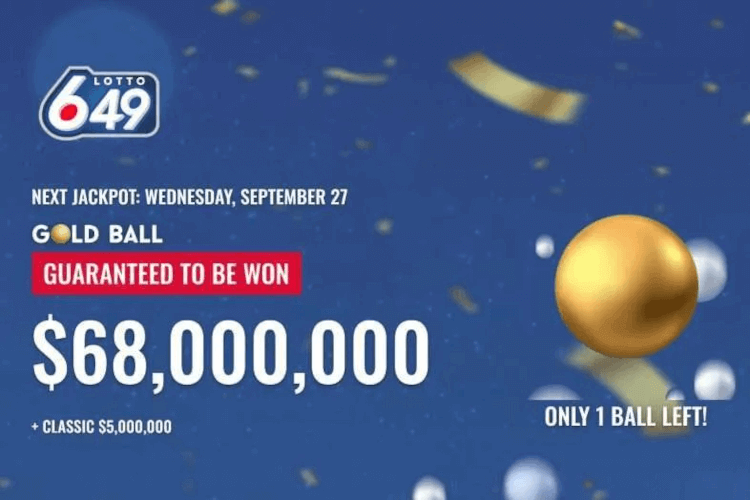# Lotto Database Blog

## Lotto 649 Gold Ball Prize of \$68 Million - What are the Odds?

Posted onThe Lotto 649 Gold Ball jackpot hit the maximum payout of \$68 million on September 27, 2023.

What are the odds the Gold Ball draw would hit the maximum?

Well, let us figure it out together.

### The Math

In total, there are 30 balls in the Gold ball draw.

There are 29 White Balls and 1 Gold Ball.

In order to have the maximum jackpot of \$68 million, the gold ball would have to be the last one drawn.

Let us look at the odds of pulling 29 white balls in a row.

The first draw is pretty easy.

The odds of pulling a white ball is 29 out of 30, or 96.67%

The white ball drawn in now placed to the side.

Now the pool has dropped to 29 balls, ie 28 white balls and 1 gold ball.

For the second draw, the odds of pulling a white ball is 28 out of 29, or 96.55%

So for the first 2 draws to be white balls only, the odds would be 96.67% x 96.55% which would give us 93.33%

You see where I am going with this?

We would have to repeat this process for all 29 white balls.

To figure out the odds with all 29 numbers, we would do the following calculation.

Odds = 29/30 x 28/29 x 27/28 x 26/27 x 25/26 . . . 4/5 x 3/4 x 2/3 x 1/2

Those familiar with math, there is a lot of cancelling which occurs.

Odds = 29/30 x 28/29 x 27/28 x 26/27 x 25/26 . . . 4/5 x 3/4 x 2/3 x 1/2.

### The Final Odds

Odds = 1/30

We are left with a simple solution of 1/30.

1 / 30 = 0.03333, which is equal to 3.33%

There is a 3.33% chance that all white balls will be drawn before the gold ball is drawn.

### It takes time.

Lotto 649 draws are twice a week, on Wednesdays and Saturdays. To have the white ball drawn 29 times in a row is not only a 3.33% chance but it also takes time.

Again some math. 29 draws / 2 draws per week = 14.5 weeks. So just over 100 days.

It already happened once, let us see how long it will take to happen again.